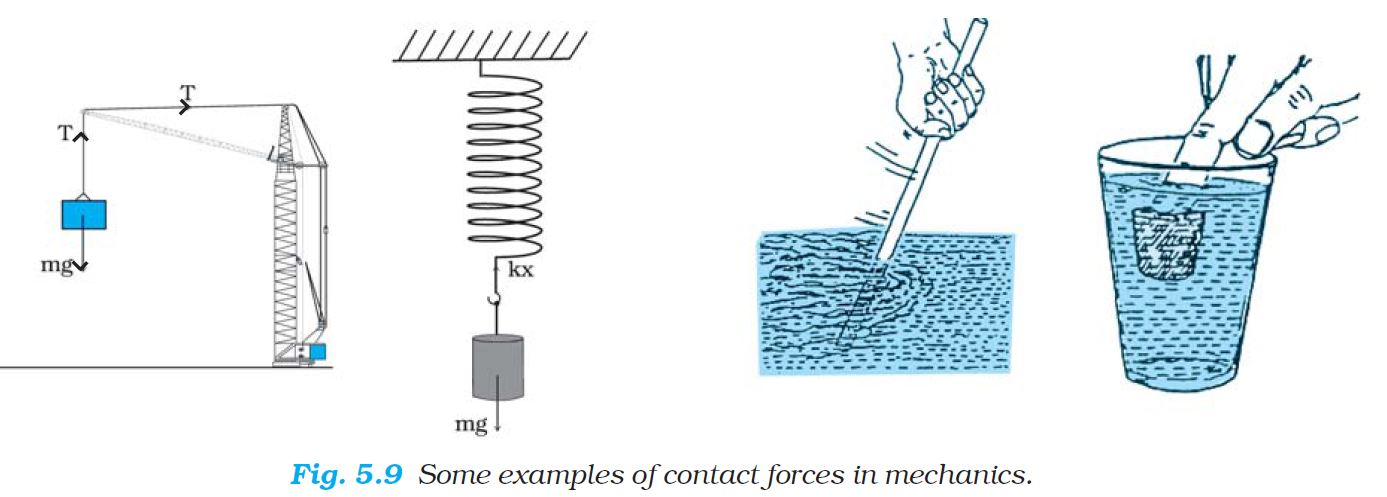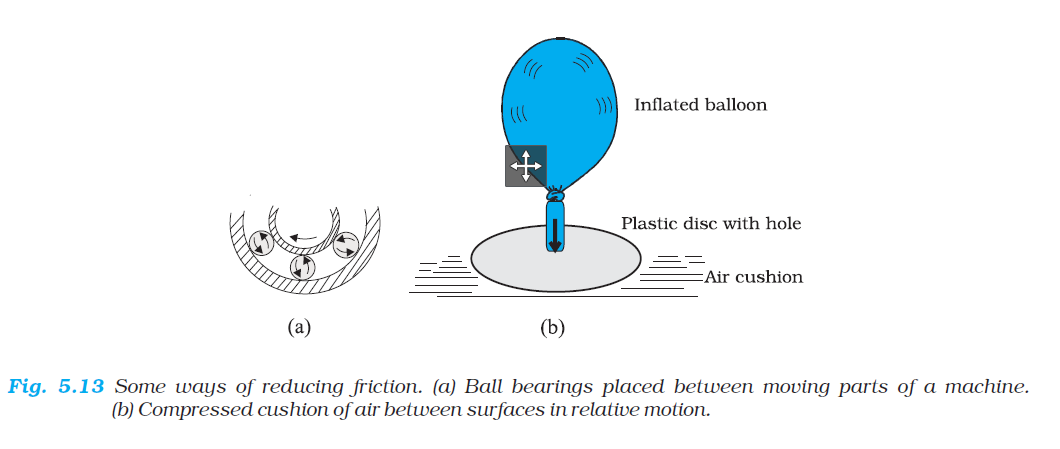Physics Different types of Friction & other contact forces

### Common Forces in Mechanicscolor{red}✍️  In mechanics, we encounter several kinds of forces. The gravitational force is, of course, all pervasive.

color{red}✍️  Every object on the earth experiences the force of gravity due to the earth. Gravity also governs the motion of celestial bodies. The gravitational force can act at a distance without the need of any intervening medium.

\color{fuchsia} {★ \mathbf\ul"Contact Forces"}

color{red}✍️  A contact force on an object arises due to contact with some other object: solid or fluid.color{red}✍️  When bodies are in contact (e.g. a book resting on a table, a system of rigid bodies connected by rods, hinges and other types of supports), there are mutual contact forces (for each pair of bodies) satisfying the third law.

color{red}✍️  The component of contact force normal to the surfaces in contact is called normal reaction.

color{red}✍️  The component parallel to the surfaces in contact is called friction.

color{red}✍️  Contact forces arise also when solids are in contact with fluids. For example, for a solid immersed in a fluid, there is an upward buoyant force equal to the weight of the fluid displaced. The viscous force, air resistance, etc are also examples of contact forces (Fig. 5.9).

\color{fuchsia} {★ \mathbf\ul"Tension Force"}

color{red}✍️  The tension force is the force that is transmitted through a string, rope, cable or wire when it is pulled tight by forces acting from opposite ends.

color{red}✍️  The tension force is directed along the length of the wire and pulls equally on the objects on the opposite ends of the wire.

color{red}✍️  For an inextensible string, the force constant is very high.

\color{fuchsia} {★ \mathbf\ul"Spring Force"}

color{red}✍️  When a spring is compressed or extended by an external force, a restoring force is generated.

color{red}✍️  This force is usually proportional to the compression or elongation (for small displacements). The spring force F is written as F = – k x where x is the displacement and k is the force constant.

color{red}✍️  The negative sign denotes that the force is opposite to the displacement from the unstretched state.

### Static Friction

color{red}✍️ Friction is that component of total contact force which acts parallel to the contact surface.

color{red}✍️ The friction force always opposes the relative slipping or tendency on relative slipping between the two contact surfaces.

color{red}✍️  When there is no relative motion between the surfaces in contact, friction is color(blue)("known as static friction.")

color{red}✍️ Direction of Static friction is such that it opposes impending motion.

color{red}✍️ When there is no applied force, there is no static friction. It comes into play the moment there is an applied force.

color{red}✍️  Static friction is an adjustable force which may have any value from zero to certain maximum value, under a given case. The maximum possible value of this static friction is known as limiting static friction.• color(red)(0<= f_s <= "Limiting static friction")

• color(red)((f_s)_(max) = mu_s N)

where color(blue)(mu_s) is a constant known as color(red)("coefficient of static friction") and color(blue)(N) is color(red)("normal reaction.")

color{red}✍️ The direction of the force of limiting friction is always opposite to the direction in which one body is on the verge of moving over the other.

color{red}✍️ The force of limiting friction is independent of the apparent contact area, so long as normal reaction between the two bodies in contact remains the same.

color{red}✍️ Limiting friction between any two bodies in contact depends on the nature of material of the surfaces in contact and their roughness and smoothness.

color{red}✍️ Its value is more than any other types of friction force.
Q 3220467311Determine the maximum acceleration of the train in which a box lying on its floor will remain stationary, given that the co-efficient of static friction between the box and the train’s floor is 0.15.Solution:

Since the acceleration of the box is due to the static friction,

ma = fs <= μ_s N = μ_s m g

i.e. a <= μ_s g

:. a_(max) = μ_s g = 0.15 x 10 m s^-2

= 1.5 m s^-2
Q 3230467312A mass of 4 kg rests on a horizontal plane. The plane is gradually inclined until at an angle theta = 15° with the horizontal, the mass just begins to slide. What is the coefficient of static friction between the block and the surface ?Solution:

The forces acting on a block of mass m at rest on an inclined plane are
(i) the weight mg acting vertically downwards
(ii) the normal force N of the plane on the block, and
(iii) the static frictional force fs opposing the impending motion. In equilibrium, the resultant of these forces must be zero. Resolving the weight mg along the two directions shown, we have

color{pink}{m g sin theta = f_s , m g cos theta = N}

As q increases, the self-adjusting frictional force f_s increases until at theta = theta_(max), achieves its maximum value, (f_s ) _(max) f = μ_s N.

Therefore,
tan theta_(max) = μ_s or  theta_(max) = tan^-1 μ_s

When q becomes just a little more than qmax , there is a small net force on the block and it begins to slide. Note that theta_(max) depends only on μ_s and is independent of the mass of the block.

For theta_(max) = 15°,

μ_s = tan 15°

= 0.27

### Kinetic Friction

color{red}✍️  Whenever there is relative motion between the surfaces in contact, friction is known as kinetic friction (f_k) which is a constant force such that

color(blue)(f_k =mu_k N)

where color(blue)(mu_k) is known as color(green)("the coefficient of kinetic friction between the surfaces")

color{red}✍️  Kinetic friction is also independent of contact area. Additionally it is also independent of relative velocity.

color{red}✍️  Experiments have revealed that coefficient of kinetic friction is always less that Coefficient of static fiction. Therefore

f_k < f_s

Q 3240467313What is the acceleration of the block and trolley system shown in a Fig. 5.12(a), if the coefficient of kinetic friction between the trolley and the surface is 0.04? What is the tension in the string? (Take g = 10 m s^-2). Neglect the mass of the string.Solution:

As the string is inextensible, and the pully is smooth, the 3 kg block and the 20 kg trolley both have same magnitude of acceleration. Applying second law to motion of the block (Fig. 5.12(b)),

30 – T = 3a

Apply the second law to motion of the trolley (Fig. 5.12(c)),

T – f_k = 20 a.

Now f_k = μ_k N,

Here μ_k = 0.04,

N = 20 x 10

= 200 N.

Thus the equation for the motion of the trolley is

T – 0.04 x 200 = 20 a Or T – 8 = 20a.

These equations give a = 22/23 m s^-2 = 0.96 m s^-2
and T = 27.1 N.

### Rolling Frictioncolor{red}✍️  A body like a ring or a sphere rolling without slipping over a horizontal plane will suffer no friction, in principle. At every instant, there is just one point of contact between the body and the plane and this point has no motion relative to the plane.color{red}✍️  In this ideal situation, color(green)("kinetic or static friction is zero") and the body should continue to roll with constant velocity.

color{red}✍️  We know, in practice, this will not happen and some resistance to motion (rolling friction) does occur, i.e. to keep the body rolling, some applied force is needed. For the same weight, rolling friction is much smaller than static or sliding friction.